In this lab we’ll investigate the probability distribution that is most central to statistics: the normal distribution. If we are confident that our data are nearly normal, that opens the door to many powerful statistical methods. Here we’ll use the graphical tools of R to assess the normality of our data and also learn how to generate random numbers from a normal distribution.

## The Data

This week we’ll be working with measurements of body dimensions. This data set contains measurements from 247 men and 260 women, most of whom were considered healthy young adults.

load("more/bdims.RData")

Let’s take a quick peek at the first few rows of the data.

head(bdims)
##   bia.di bii.di bit.di che.de che.di elb.di wri.di kne.di ank.di sho.gi
## 1   42.9   26.0   31.5   17.7   28.0   13.1   10.4   18.8   14.1  106.2
## 2   43.7   28.5   33.5   16.9   30.8   14.0   11.8   20.6   15.1  110.5
## 3   40.1   28.2   33.3   20.9   31.7   13.9   10.9   19.7   14.1  115.1
## 4   44.3   29.9   34.0   18.4   28.2   13.9   11.2   20.9   15.0  104.5
## 5   42.5   29.9   34.0   21.5   29.4   15.2   11.6   20.7   14.9  107.5
## 6   43.3   27.0   31.5   19.6   31.3   14.0   11.5   18.8   13.9  119.8
##   che.gi wai.gi nav.gi hip.gi thi.gi bic.gi for.gi kne.gi cal.gi ank.gi
## 1   89.5   71.5   74.5   93.5   51.5   32.5   26.0   34.5   36.5   23.5
## 2   97.0   79.0   86.5   94.8   51.5   34.4   28.0   36.5   37.5   24.5
## 3   97.5   83.2   82.9   95.0   57.3   33.4   28.8   37.0   37.3   21.9
## 4   97.0   77.8   78.8   94.0   53.0   31.0   26.2   37.0   34.8   23.0
## 5   97.5   80.0   82.5   98.5   55.4   32.0   28.4   37.7   38.6   24.4
## 6   99.9   82.5   80.1   95.3   57.5   33.0   28.0   36.6   36.1   23.5
##   wri.gi age  wgt   hgt sex
## 1   16.5  21 65.6 174.0   1
## 2   17.0  23 71.8 175.3   1
## 3   16.9  28 80.7 193.5   1
## 4   16.6  23 72.6 186.5   1
## 5   18.0  22 78.8 187.2   1
## 6   16.9  21 74.8 181.5   1

You’ll see that for every observation we have 25 measurements, many of which are either diameters or girths. A key to the variable names can be found at http://www.openintro.org/stat/data/bdims.php, but we’ll be focusing on just three columns to get started: weight in kg (wgt), height in cm (hgt), and sex (1 indicates male, 0 indicates female).

Since males and females tend to have different body dimensions, it will be useful to create two additional data sets: one with only men and another with only women.

mdims <- subset(bdims, sex == 1)
fdims <- subset(bdims, sex == 0)
1. Make a histogram of men’s heights and a histogram of women’s heights. How would you compare the various aspects of the two distributions?
hist(mdims$hgt)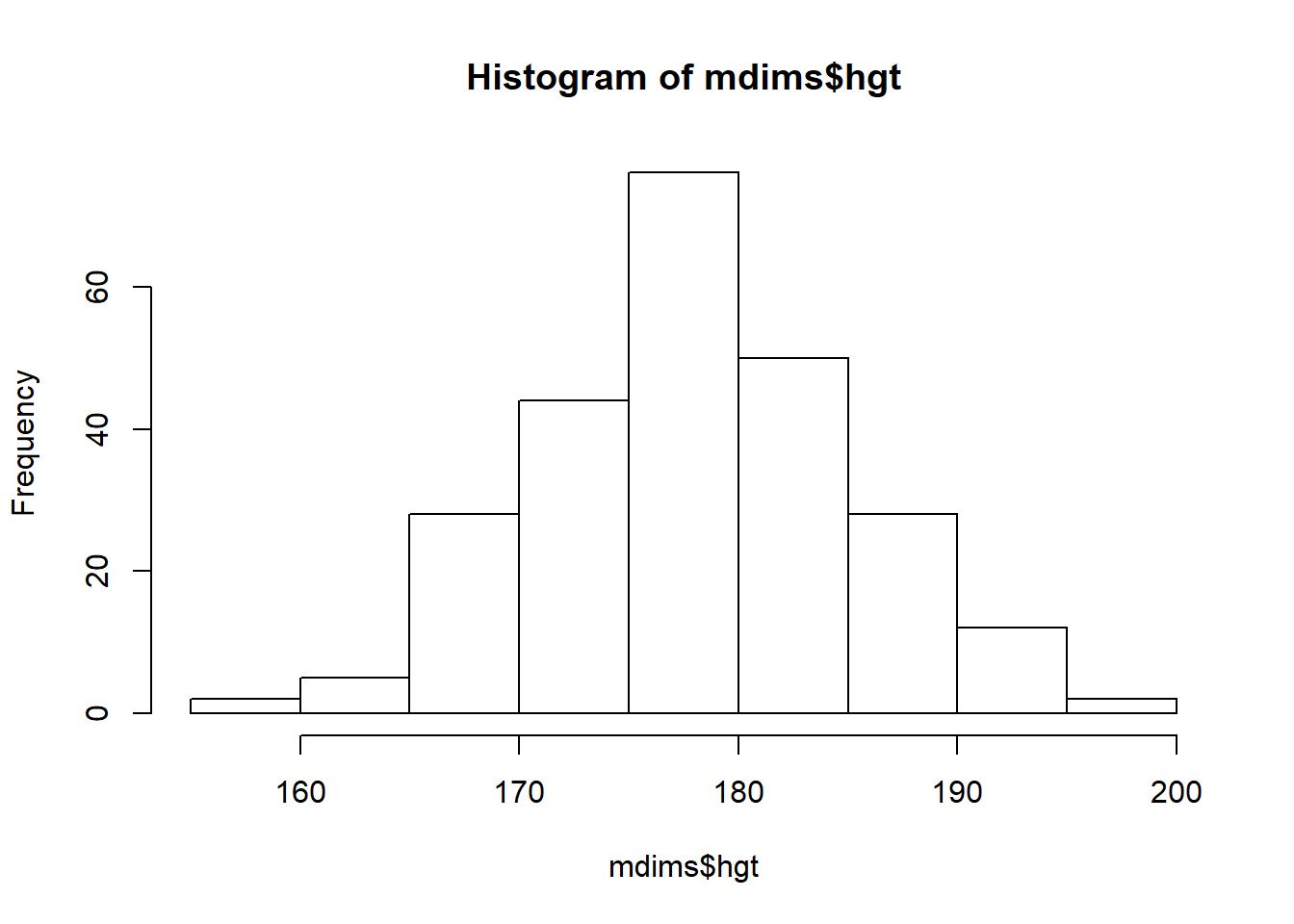hist(fdims$hgt)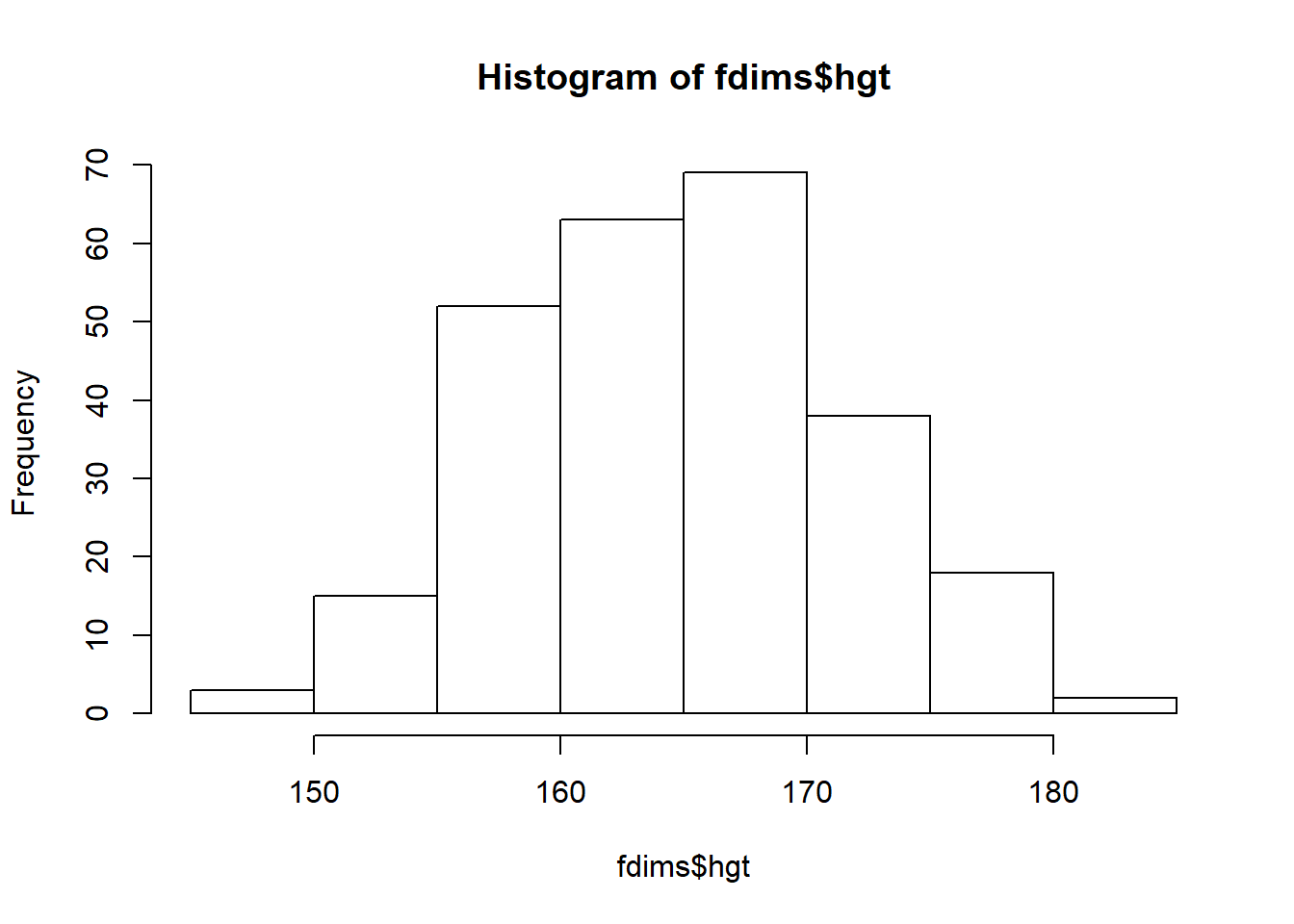## The normal distribution

In your description of the distributions, did you use words like bell-shaped or normal? It’s tempting to say so when faced with a unimodal symmetric distribution.

To see how accurate that description is, we can plot a normal distribution curve on top of a histogram to see how closely the data follow a normal distribution. This normal curve should have the same mean and standard deviation as the data. We’ll be working with women’s heights, so let’s store them as a separate object and then calculate some statistics that will be referenced later.

fhgtmean <- mean(fdims$hgt) fhgtsd <- sd(fdims$hgt)

Next we make a density histogram to use as the backdrop and use the lines function to overlay a normal probability curve. The difference between a frequency histogram and a density histogram is that while in a frequency histogram the heights of the bars add up to the total number of observations, in a density histogram the areas of the bars add up to 1. The area of each bar can be calculated as simply the height times the width of the bar. Using a density histogram allows us to properly overlay a normal distribution curve over the histogram since the curve is a normal probability density function. Frequency and density histograms both display the same exact shape; they only differ in their y-axis. You can verify this by comparing the frequency histogram you constructed earlier and the density histogram created by the commands below.

hist(fdims$hgt, probability = TRUE,ylim = c(0, 0.06)) x <- 140:190 y <- dnorm(x = x, mean = fhgtmean, sd = fhgtsd) lines(x = x, y = y, col = "blue")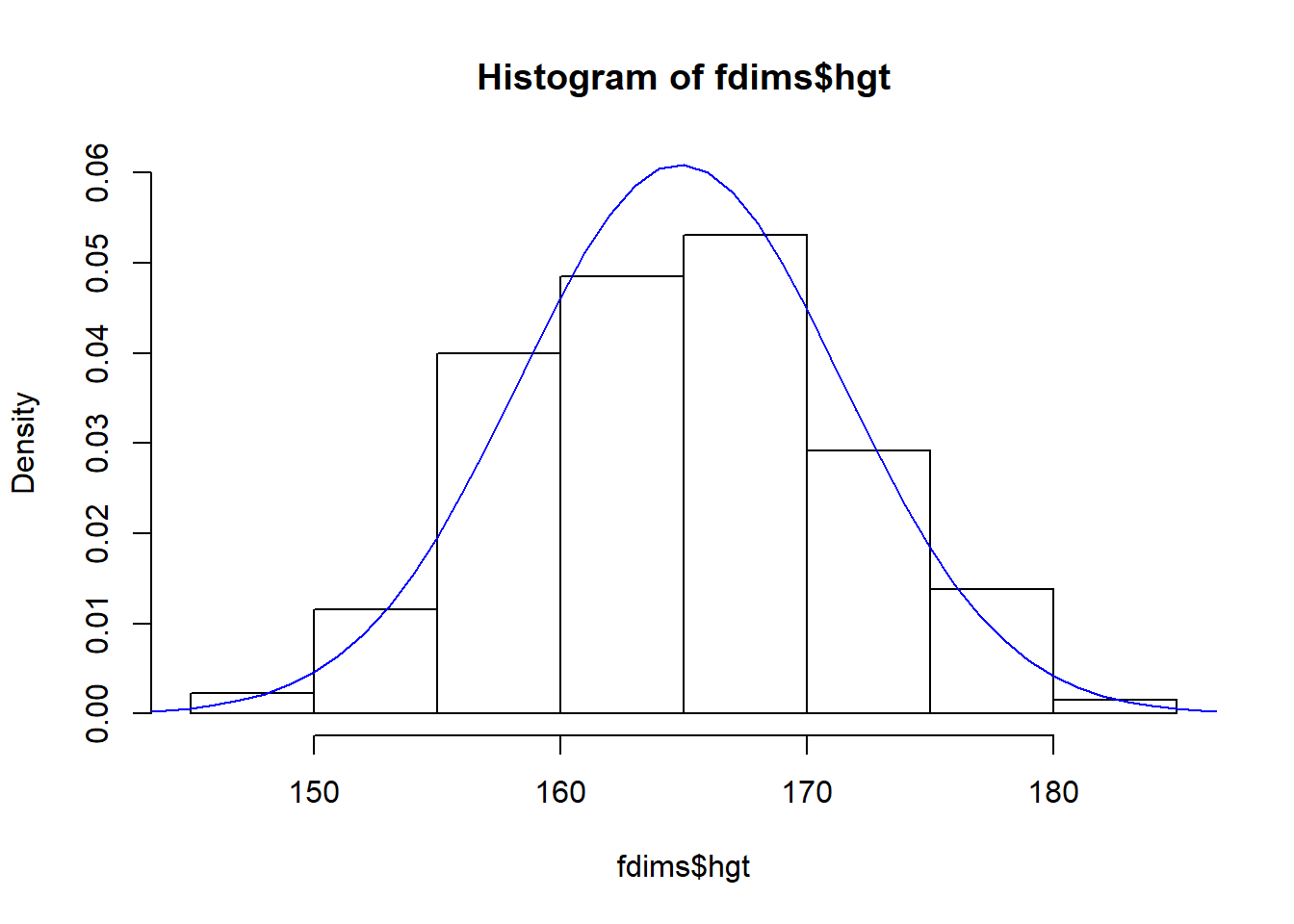After plotting the density histogram with the first command, we create the x- and y-coordinates for the normal curve. We chose the x range as 140 to 190 in order to span the entire range of fheight. To create y, we use dnorm to calculate the density of each of those x-values in a distribution that is normal with mean fhgtmean and standard deviation fhgtsd. The final command draws a curve on the existing plot (the density histogram) by connecting each of the points specified by x and y. The argument col simply sets the color for the line to be drawn. If we left it out, the line would be drawn in black. The top of the curve is cut off because the limits of the x- and y-axes are set to best fit the histogram. To adjust the y-axis you can add a third argument to the histogram function: ylim = c(0, 0.06). 1. Based on the this plot, does it appear that the data follow a nearly normal distribution? ### The data does appear to closely follow a nearly normal distribution. ## Evaluating the normal distribution Eyeballing the shape of the histogram is one way to determine if the data appear to be nearly normally distributed, but it can be frustrating to decide just how close the histogram is to the curve. An alternative approach involves constructing a normal probability plot, also called a normal Q-Q plot for “quantile-quantile”. qqnorm(fdims$hgt)
qqline(fdims$hgt)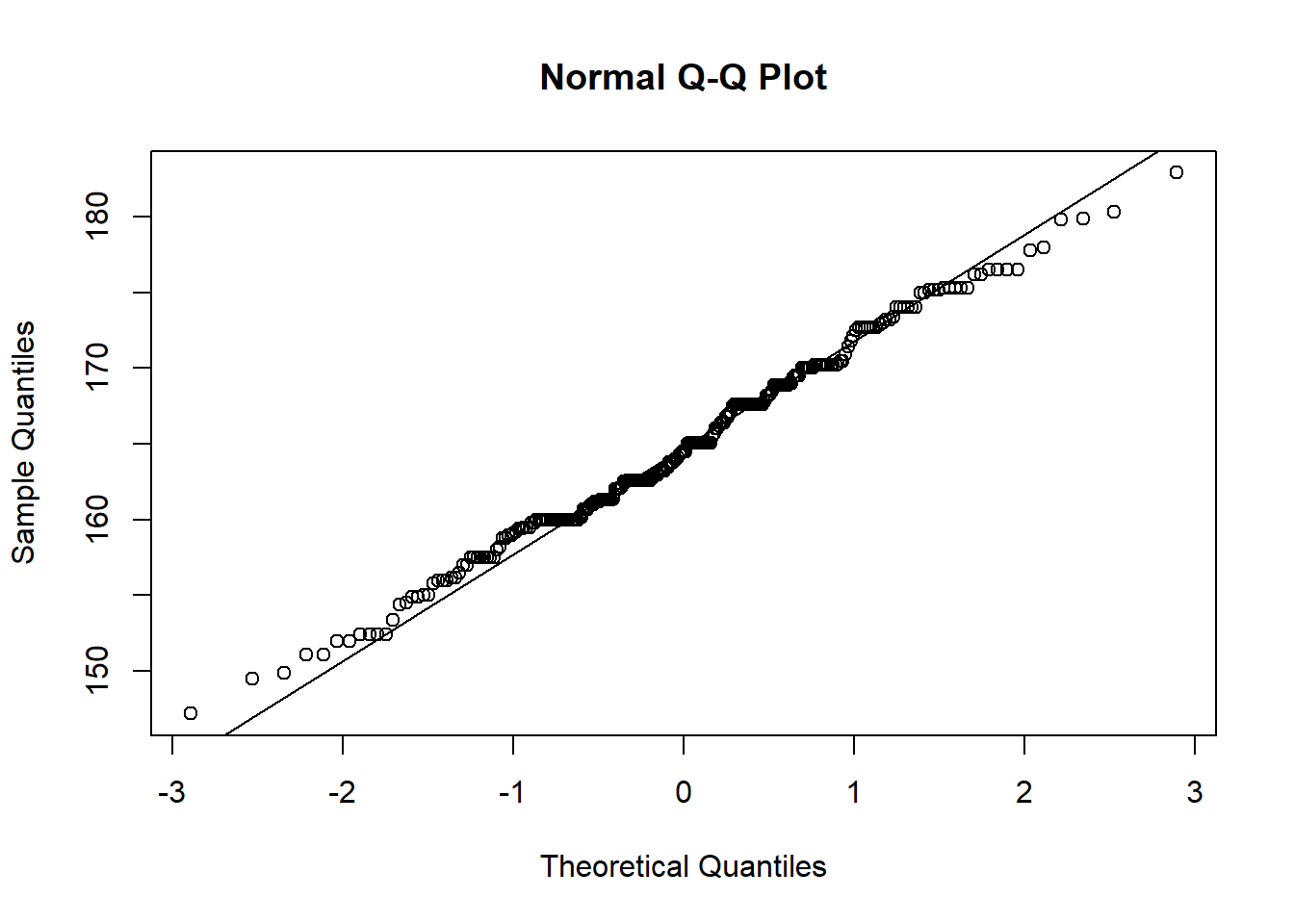A data set that is nearly normal will result in a probability plot where the points closely follow the line. Any deviations from normality leads to deviations of these points from the line. The plot for female heights shows points that tend to follow the line but with some errant points towards the tails. We’re left with the same problem that we encountered with the histogram above: how close is close enough? A useful way to address this question is to rephrase it as: what do probability plots look like for data that I know came from a normal distribution? We can answer this by simulating data from a normal distribution using rnorm. sim_norm <- rnorm(n = length(fdims$hgt), mean = fhgtmean, sd = fhgtsd)

The first argument indicates how many numbers you’d like to generate, which we specify to be the same number of heights in the fdims data set using the length function. The last two arguments determine the mean and standard deviation of the normal distribution from which the simulated sample will be generated. We can take a look at the shape of our simulated data set, sim_norm, as well as its normal probability plot.

1. Make a normal probability plot of sim_norm. Do all of the points fall on the line? How does this plot compare to the probability plot for the real data?
qqnorm(sim_norm)
qqline(fdims$hgt)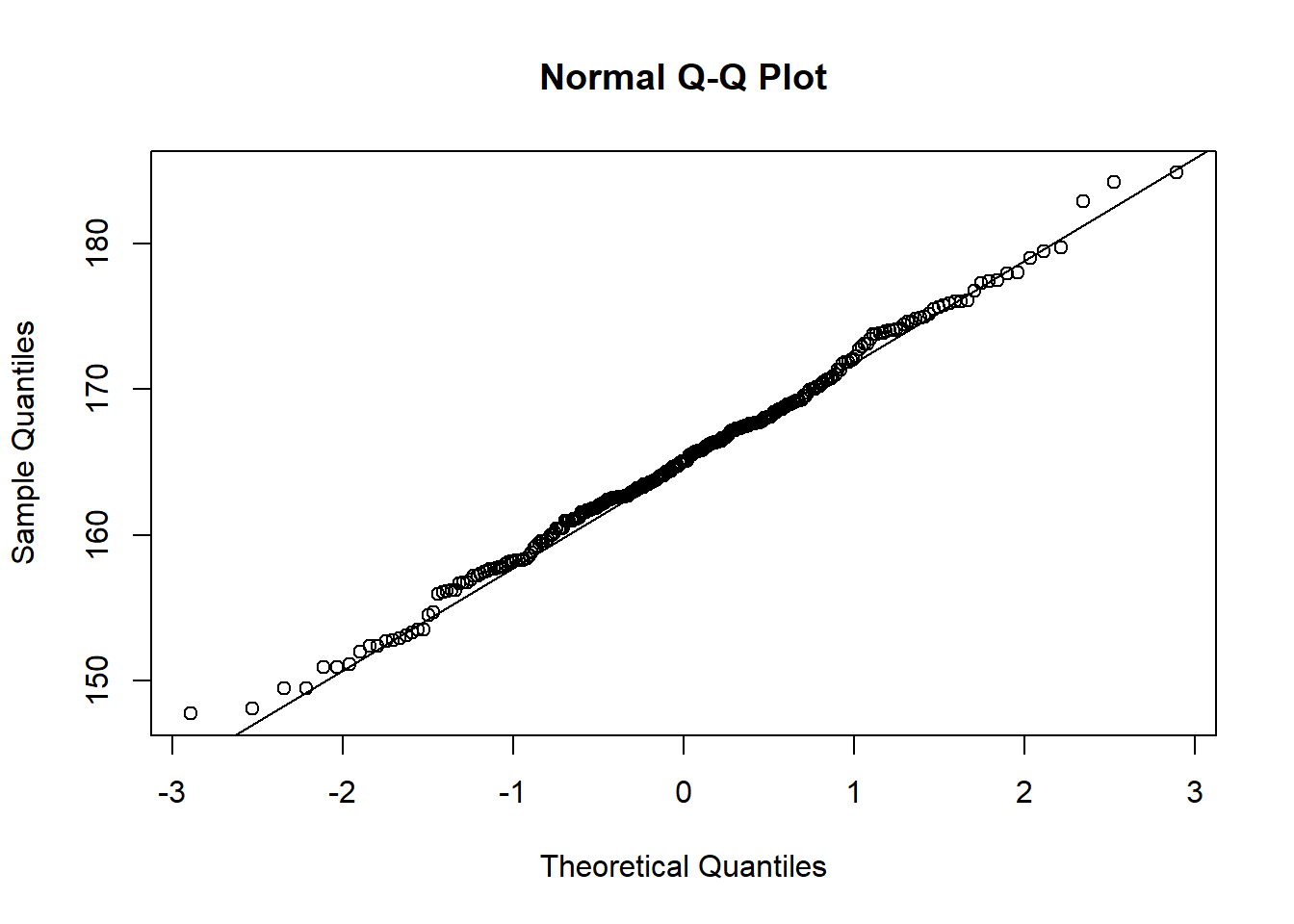### The distribution is extremely similar to what we saw for fdims$hgt, so it seems that a simulated normal distribution deviates from the line just as much as our data for female heights, suggesting the female height data is normally distributed.

Even better than comparing the original plot to a single plot generated from a normal distribution is to compare it to many more plots using the following function. It may be helpful to click the zoom button in the plot window.

qqnormsim(fdims$hgt)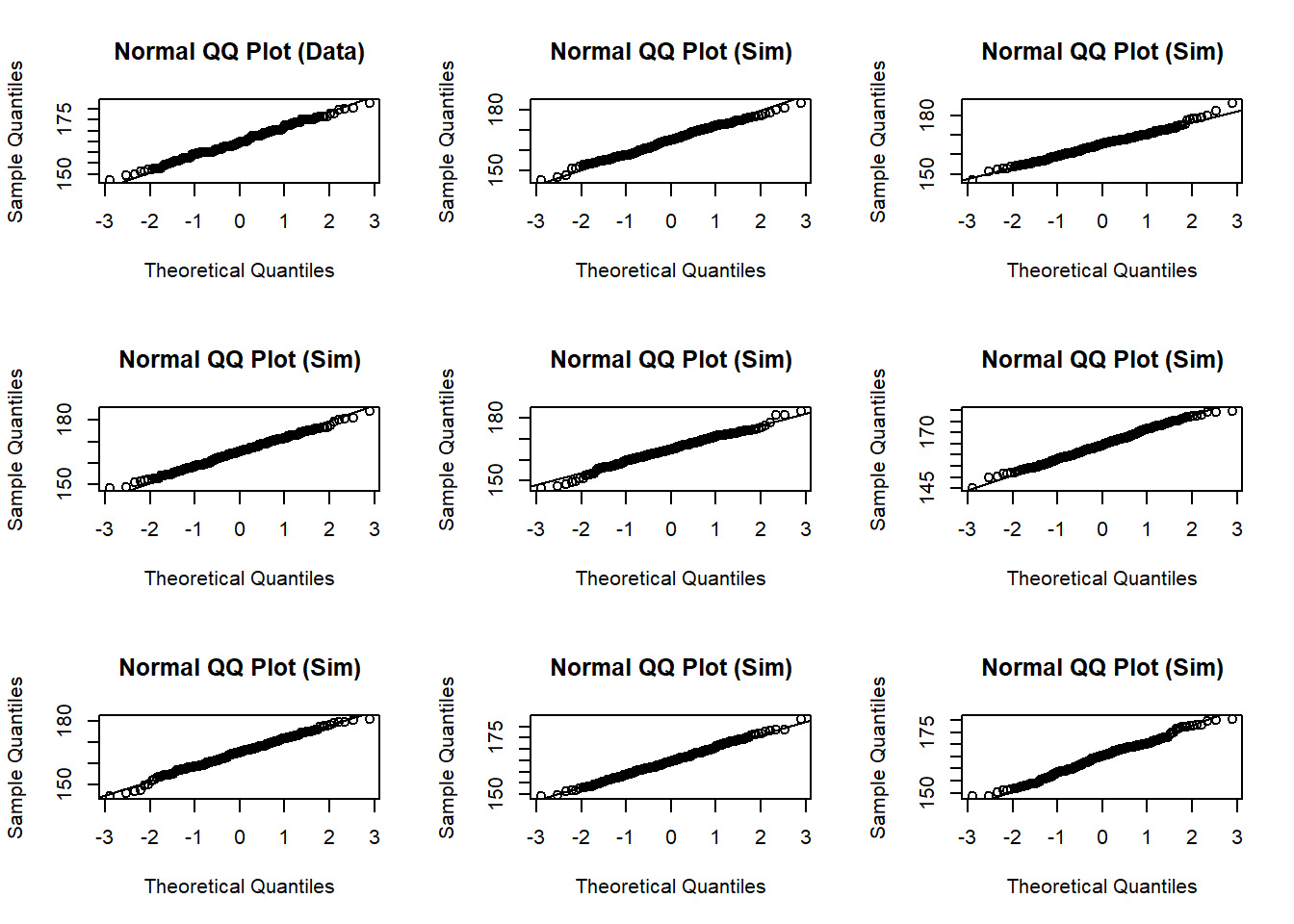1. Does the normal probability plot for fdims$hgt look similar to the plots created for the simulated data? That is, do plots provide evidence that the female heights are nearly normal?

### The significant similarity with the simulated plots indicates that the female heights are nearly normal.

1. Using the same technique, determine whether or not female weights appear to come from a normal distribution.
qqnorm(fdims$wgt) qqline(fdims$wgt)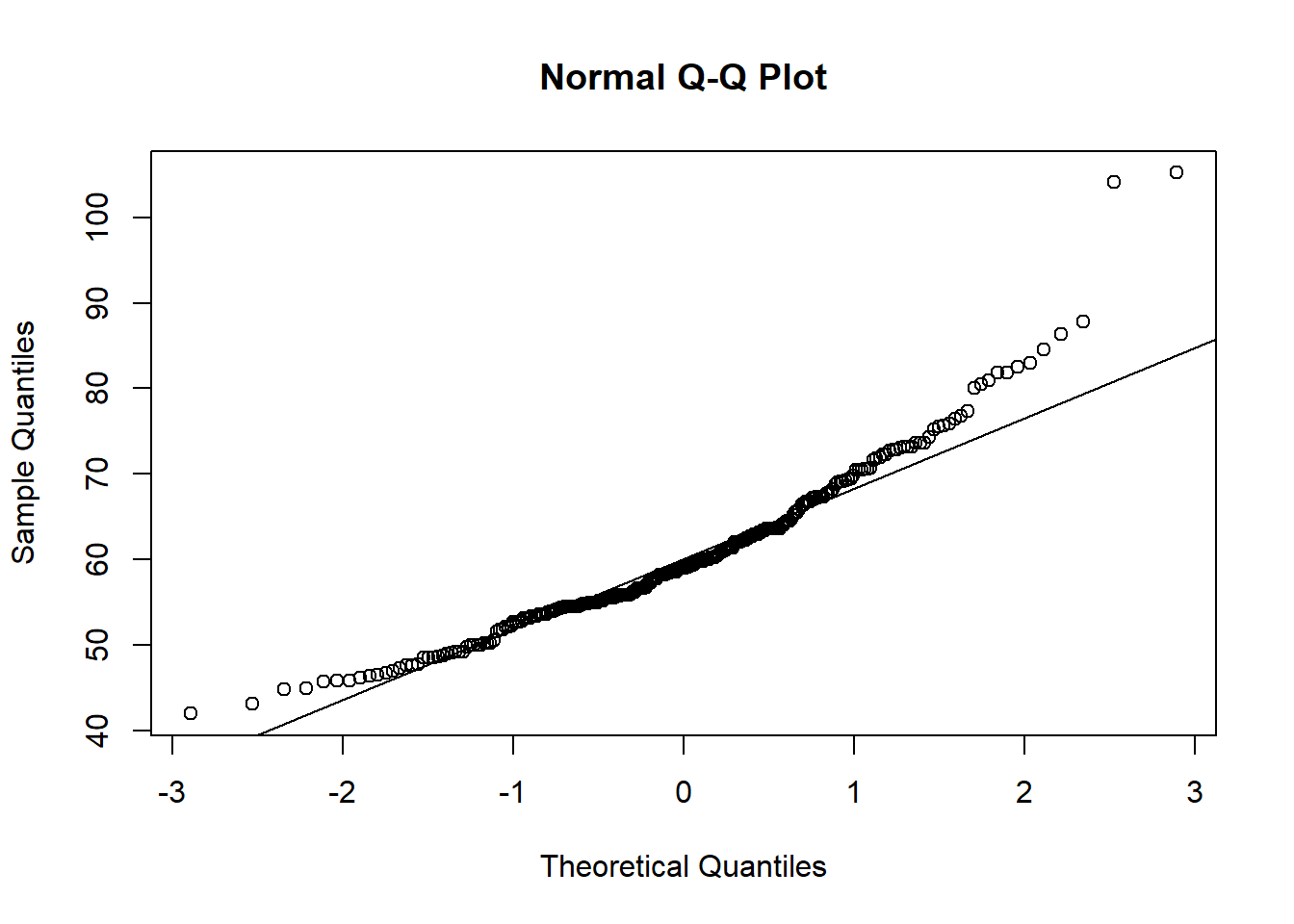fwgtsd   <- sd(fdims$wgt) 1 - pnorm(q=65, mean = fwgtmean, sd = fwgtsd) ##  0.3236397 sum(fdims$wgt > 65) / length(fdims$wgt) ##  0.2615385 ### The height data has a much closer agreement with the normal distribution, as indicated in the previous answers. ## On Your Own • Now let’s consider some of the other variables in the body dimensions data set. Using the figures at the end of the exercises, match the histogram to its normal probability plot. All of the variables have been standardized (first subtract the mean, then divide by the standard deviation), so the units won’t be of any help. If you are uncertain based on these figures, generate the plots in R to check. a. The histogram for female biiliac (pelvic) diameter (bii.di) belongs to normal probability plot letter B. b. The histogram for female elbow diameter (elb.di) belongs to normal probability plot letter C. c. The histogram for general age (age) belongs to normal probability plot letter D. d. The histogram for female chest depth (che.de) belongs to normal probability plot letter A. • Note that normal probability plots C and D have a slight stepwise pattern. Why do you think this is the case? ### Plot D corresponds to age, which would have been reported in discrete integers, explaining th estep-like nature of th eplot. Plot C • As you can see, normal probability plots can be used both to assess normality and visualize skewness. Make a normal probability plot for female knee diameter (kne.di). Based on this normal probability plot, is this variable left skewed, symmetric, or right skewed? Use a histogram to confirm your findings. qqnorm(fdims$kne.di)
qqline(fdims$kne.di)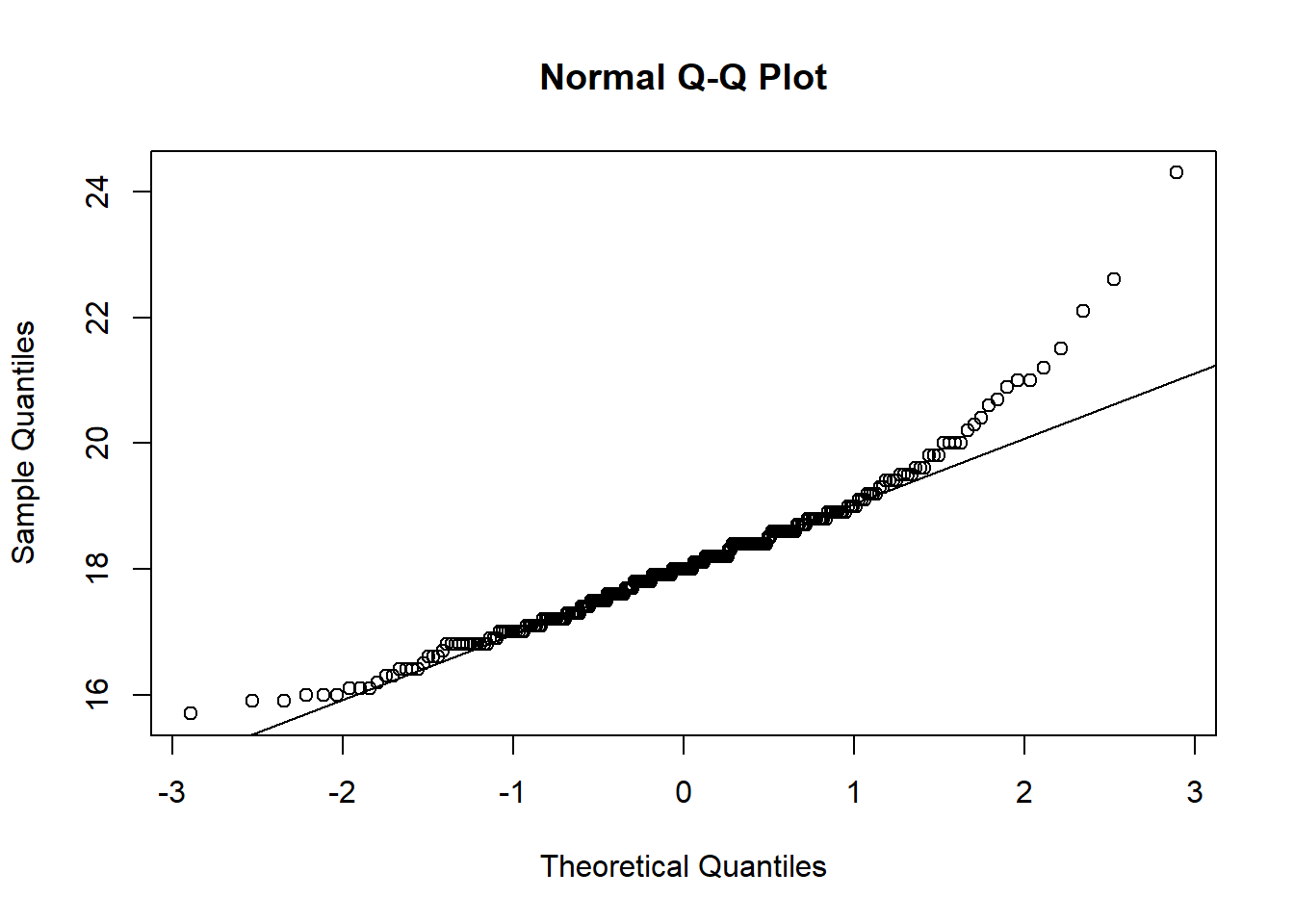#Based on the normal probability plot, this is a right-tailed plot. hist(fdims$kne.di)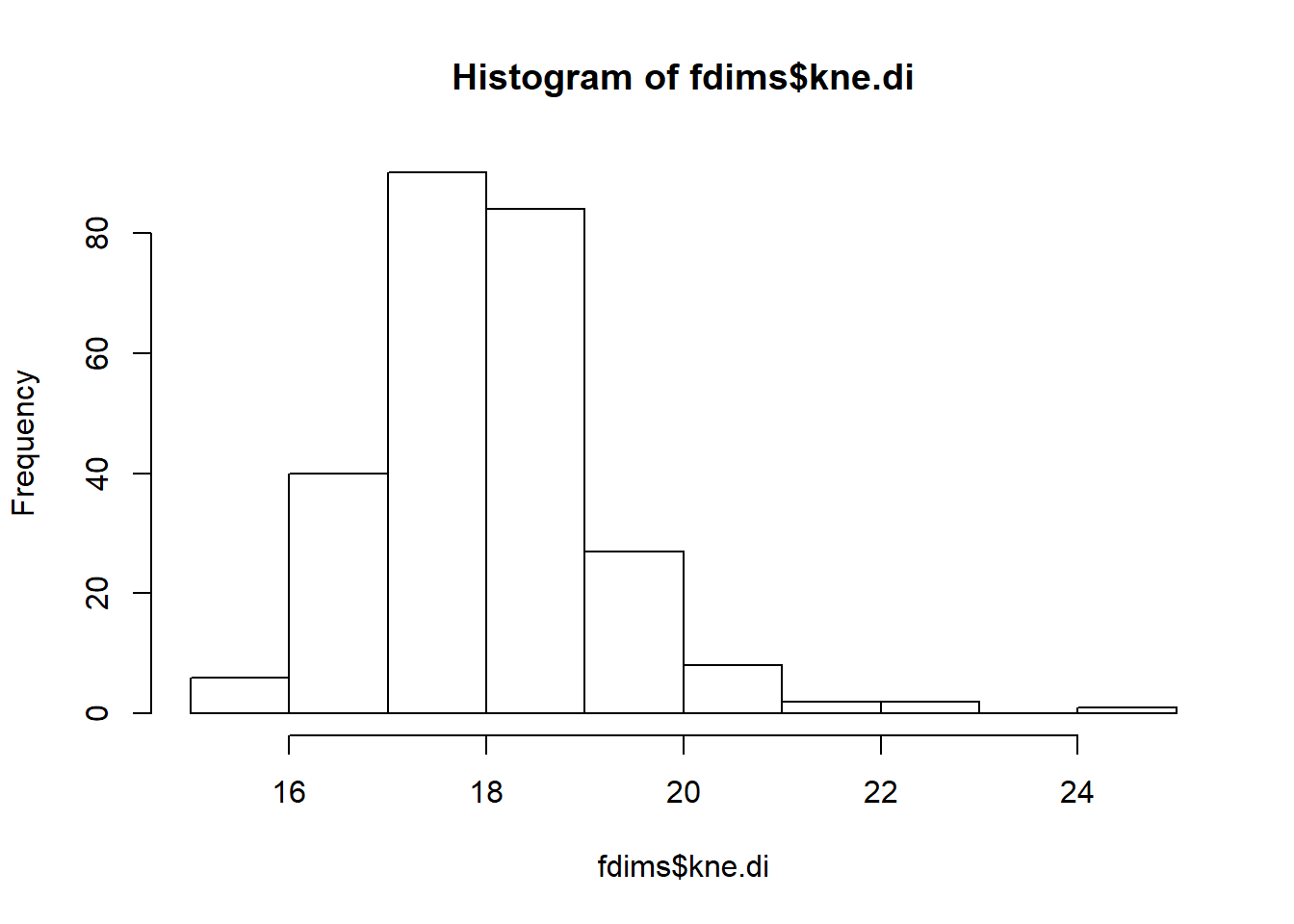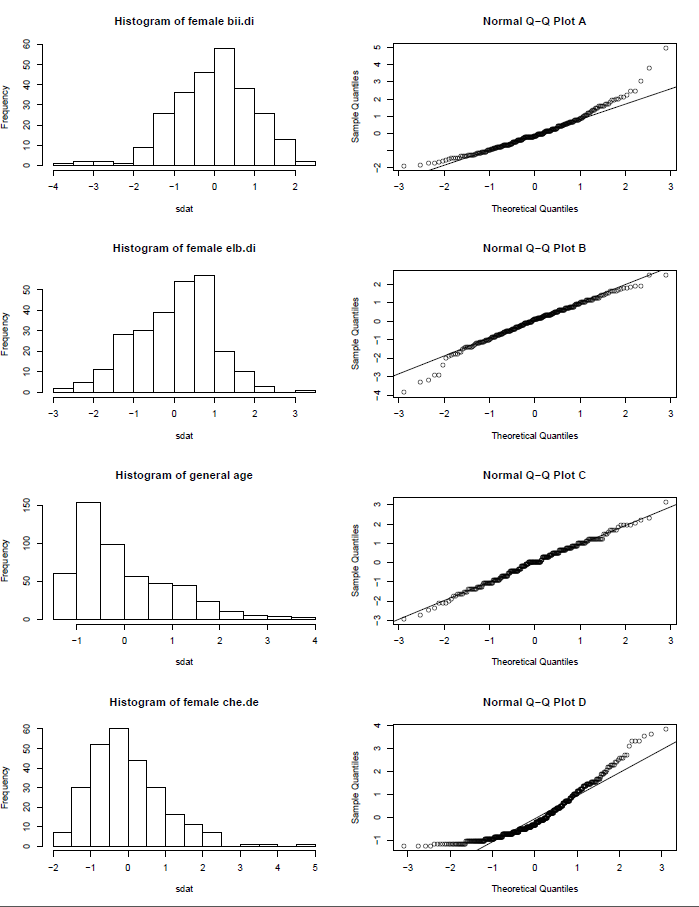histQQmatch